GMAT Math : Circles

Example Questions

1 2 3 4 5 6 7 8 9 10 12 Next →

Example Question #6 : Chords

The arc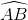of a circle measuresand has length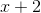. Give the length of the chord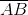.Explanation:

The figure referenced is below.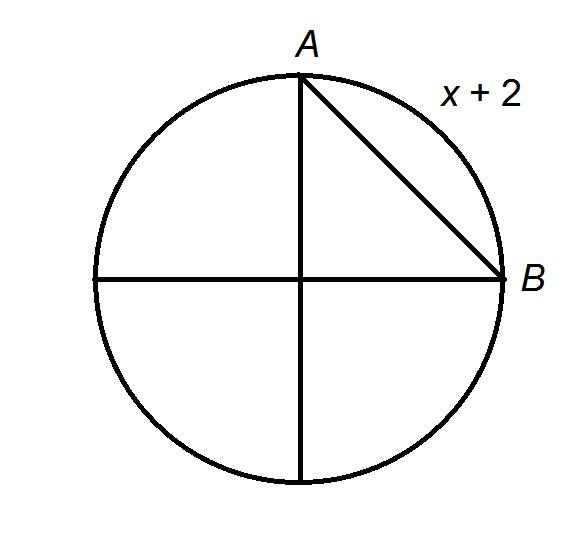The arc is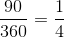of the circle, so the circumference of the circle is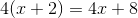.

The radius is this circumference divided by, or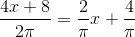.is, consequently, the hypotenuse of an isosceles right triangle with leg length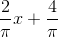; by the 45-45-90 Triangle Theorem, its length istimes this, or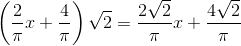1 2 3 4 5 6 7 8 9 10 12 Next →

All GMAT Math Resources